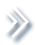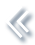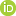####ArchivesReview Articles

## Efficient estimation of smoothing spline with exact shape constraints

Vincent Chan ,Kam-Wah Tsui ,Yanran Wei ,

b Department of Statistics, Virginia Tech, Blacksburg, VA, USAZhiyang Zhang ,

b Department of Statistics, Virginia Tech, Blacksburg, VA, USAXinwei Deng

b Department of Statistics, Virginia Tech, Blacksburg, VA, USAxdeng@vt.edu

Pages 55-69 | Received 14 Feb. 2019, Accepted 24 Jan. 2020, Published online: 07 Feb. 2020,
• Abstract
• References
• Citations

ABSTRACT

Smoothing spline is a popular method in non-parametric function estimation. For the analysis of data from real applications, specific shapes on the estimated function are often required to ensure the estimated function undeviating from the domain knowledge. In this work, we focus on constructing the exact shape constrained smoothing spline with efficient estimation. The ‘exact’ here is referred as to impose the shape constraint on an infinite set such as an interval in one-dimensional case. Thus the estimation becomes a so-called semi-infinite optimisation problem with an infinite number of constraints. The proposed method is able to establish a sufficient and necessary condition for transforming the exact shape constraints to a finite number of constraints, leading to efficient estimation of the shape constrained functions. The performance of the proposed methods is evaluated by both simulation and real case studies.

## References

1. Brezger, A., & Steiner, W. J. (2003). Monotonic regression based on Bayesian P-splines: An application to estimating price response functions from store-level scanner data. Tech. rep., Discussion paper//Sonderforschungsbereich 386 der Ludwig-Maximilians-Universität München. [Google Scholar]
2. Curry, H. B., & Schoenberg, I. J. (1966). On Pólya frequency functions IV: The fundamental spline functions and their limits. Journal D'analyse Mathématique17, 71–107. doi: 10.1007/BF02788653 [Crossref][Web of Science ®], [Google Scholar]
3. Dai, X., & Chien, P. (2017). Minimax optimal rates of estimation in functional anova models with derivatives. arXiv:1706.00850. [Google Scholar]
4. Delecroix, M., & Thomas-Agnan, C. (2000). Spline and Kernel regression under shape restrictions. In Smoothing and Regression: Approaches, Computation, and Application (pp. 109–133). [Crossref], [Google Scholar]
5. Dierckx, I. P (1980). Algorithm/algorithmus 42 an algorithm for cubic spline fitting with convexity constraints. Computing24, 349–371. doi: 10.1007/BF02237820 [Crossref][Web of Science ®], [Google Scholar]
6. Ducharme, G. R., & Fontez, B. (2004). A smooth test of goodness-of-fit for growth curves and monotonic nonlinear regression models. Biometrics60, 977–986. doi: 10.1111/j.0006-341X.2004.00253.x [Crossref][Web of Science ®], [Google Scholar]
7. Fan, J., & Gijbels, I. (1996). Local polynomial modelling and its applications: Monographs on statistics and applied probability. New York: CRC Press. [Google Scholar]
8. Green, P. J. (1987). Penalized likelihood for general semi-parametric regression models. International Statistical Review / Revue Internationale de Statistique55(3), 245. [Web of Science ®], [Google Scholar]
9. Green, P. J., & Silverman, B. W. (1993). Nonparametric regression and generalized linear models: A roughness penalty approach. New York: CRC Press. [Crossref], [Google Scholar]
10. Hastie, T., Tibshirani, R., & Friedman, J, The elements of statistical learning, Springer series in statistics Springer (Vol. 1). Berlin, 2001. [Crossref], [Google Scholar]
11. He, X., & Shi, P. (1998). Monotone B-spline smoothing. Journal of the American Statistical Association93, 643–650. [Taylor & Francis Online][Web of Science ®], [Google Scholar]
12. Kelly, C., & Rice, J. (1990). Monotone smoothing with application to dose-response curves and the assessment of synergism. Biometrics46(4), 1071–1085. doi: 10.2307/2532449 [Crossref][Web of Science ®], [Google Scholar]
13. Liao, X., & Meyer, M. C. (2017). Change-point estimation using shape-restricted regression splines. Journal of Statistical Planning and Inference188, 8–21. doi: 10.1016/j.jspi.2017.03.007 [Crossref][Web of Science ®], [Google Scholar]
14. Lichman, M. (2013), UCI machine learning repository. [Google Scholar]
15. Mammen, E., & Thomas-Agnan, C. (1999). Smoothing splines and shape restrictions. Scandinavian Journal of Statistics26, 239–252. doi: 10.1111/1467-9469.00147 [Crossref][Web of Science ®], [Google Scholar]
16. Matzkin, R. L. (1991). Semiparametric estimation of monotone and concave utility functions for polychotomous choice models. Econometrica: Journal of the Econometric Society59,1315–1327. doi: 10.2307/2938369 [Crossref][Web of Science ®], [Google Scholar]
17. Meyer, M. C. (2008). Inference using shape-restricted regression splines. The Annals of Applied Statistics2, 1013–1033. doi: 10.1214/08-AOAS167 [Crossref][Web of Science ®], [Google Scholar]
18. Meyer, M. C. (2012). Constrained penalized splines. Canadian Journal of Statistics40, 190–206. doi: 10.1002/cjs.10137 [Crossref][Web of Science ®], [Google Scholar]
19. Meyer, M. C. (2018). Constrained partial linear regression splines. Statistica Sinica28, 277–292. [Web of Science ®], [Google Scholar]
20. Nagahara, M., & Martin, C. F. (2013). Monotone smoothing splines using general linear systems. Asian Journal of Control15, 461–468. doi: 10.1002/asjc.557 [Crossref][Web of Science ®], [Google Scholar]
21. Ramsay, J. O. (1988). Monotone regression splines in action. Statistical Science3,425–441. doi: 10.1214/ss/1177012761 [Crossref], [Google Scholar]
22. Shor, N. Z., & Zhurbenko, N. (1971). The minimization method using space dilatation in direction of difference of two sequential gradients. Kibernetika3, 51–59. [Google Scholar]
23. Turlach, B. A. (2005). Shape constrained smoothing using smoothing splines. Computational Statistics20, 81–104. doi: 10.1007/BF02736124 [Crossref][Web of Science ®], [Google Scholar]
24. Utreras, F. I. (1985). Smoothing noisy data under monotonicity constraints existence, characterization and convergence rates. Numerische Mathematik47, 611–625. doi: 10.1007/BF01389460 [Crossref][Web of Science ®], [Google Scholar]
25. Villalobos, M., & Wahba, G. (1987). Inequality-constrained multivariate smoothing splines with application to the estimation of posterior probabilities. Journal of the American Statistical Association82, 239–248. doi: 10.1080/01621459.1987.10478426 [Taylor & Francis Online][Web of Science ®], [Google Scholar]
26. Wahba, G. (1990). Spline models for observational data. Philadelphia, Pennsylvania: Siam. [Crossref], [Google Scholar]
27. Wand, M., & Jones, M.. (1995), Kernel smoothing. Vol. 60 of Monographs on statistics and applied probability. [Crossref], [Google Scholar]
28. Wand, M. P., & Ormerod, J. T. (2008). On Semiparametric regression with O'sullivan penalized splines. Australian & New Zealand Journal of Statistics50(2), 179–198. doi: 10.1111/j.1467-842X.2008.00507.x [Crossref][Web of Science ®], [Google Scholar]
29. Wang, X., & Li, F. (2008). Isotonic smoothing spline regression. Journal of Computational and Graphical Statistics17, 21–37. doi: 10.1198/106186008X285627 [Taylor & Francis Online][Web of Science ®], [Google Scholar]
30. Zeng, L., Deng, X., & Yang, J. (2016). Constrained hierarchical modeling of degradation data in tissue-engineered scaffold fabrication. IIE Transactions48, 16–33. doi: 10.1080/0740817X.2015.1019164 [Taylor & Francis Online][Web of Science ®], [Google Scholar]
31. Zhang, D., Lin, X., Raz, J., & Sowers, M. (1998). Semiparametric stochastic mixed models for longitudinal data. Journal of the American Statistical Association93(442), 710. doi: 10.1080/01621459.1998.10473723 [Taylor & Francis Online][Web of Science ®], [Google Scholar]